Mathematical and Physical Journal
for High Schools
Issued by the MATFUND Foundation
 Already signed up? New to KöMaL?

# KöMaL Problems in Informatics, February 2011

Show/hide problems of signs:## Problems with sign 'I'

Deadline expired on March 10, 2011.

I. 259. Recursion is a tool often used both in mathematics and in informatics. It can appear in different stages during the solution of a problem, for example, in the specification part, in algorithmic planning, or during the coding in the programming language.

One typically uses a function to give the specification. This function effectively establishes a connection between the input and the output. Let us first consider the power function for example. A possible definition is the following: $\displaystyle x^{n}=x\cdot x^{n-1}$ and $\displaystyle x^{0}=1$, or, in other words,

$\displaystyle \mathop{\rm power}\, (x,n)= \begin{cases} 1 & \text{if \ } n=0, \\ x \cdot \mathop{\rm power}\, (x,n-1) & \text{if \ } n>0. \end{cases}$

In this way, the computation of $\displaystyle 5^{3}$ is reduced to $\displaystyle 5\cdot 5^{2}$, that is to $\displaystyle 5^{2}$, then to $\displaystyle 5^{1}$, finally, to $\displaystyle 5^{0}$, which is defined separately. The above task can be solved effectively with a recursive algorithm: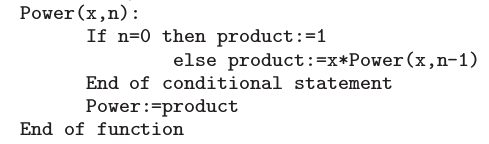In functional programming languages, like ImagineLogo, coding simply follows from the above: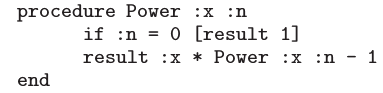Non-recursive languages, like Pascal, may also allow this coding to be implemented: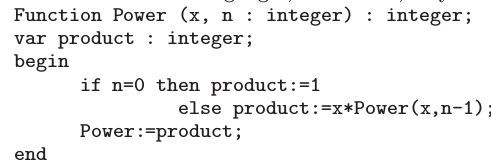Algorithms and programs defined iteratively can be rewritten in a recursive fashion. Sequences and conditionals, as the most important algorithmic blocks, can be transformed without any difficulty or modification. Loops however require some revision to make them recursive. The following scheme illustrates how to reformulate a pre-test loop (that is, a loop in which the loop test is evaluated before the body of the loop):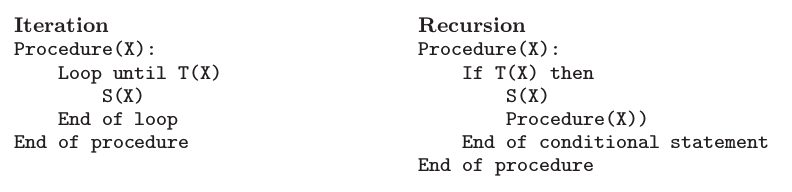Example. Create the sequence of natural numbers between $\displaystyle A$ and $\displaystyle B$ ($\displaystyle 1\le A,B\le 100$). In the solution below, the procedure Előállít(5, 10, Sor, 1) puts the sequence 5 6 7 8 9 10 into the array Sor.

I. Iterative solution: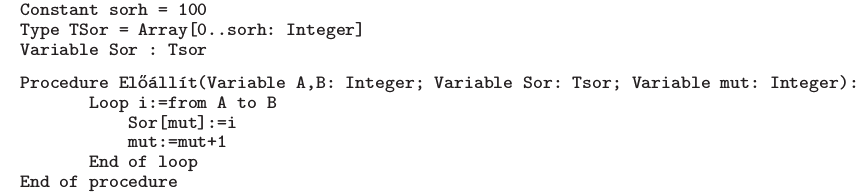II. Recursive solution: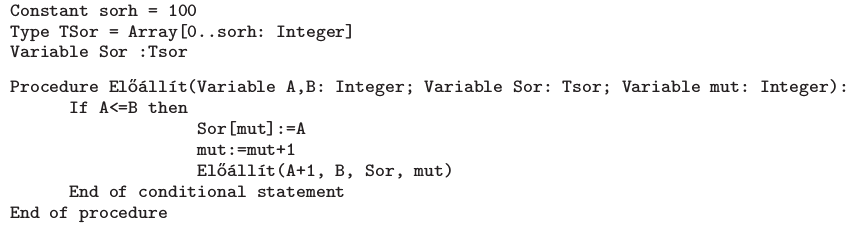Your task is to create a program that produces the following sequences without using any loop.

$\displaystyle a)$ Write a function to create a mountain of numbers'' of height $\displaystyle N$ ($\displaystyle N\ge 1$). Example: mountain 5 Result: 1 2 3 4 5 4 3 2 1;

$\displaystyle b)$ Write a function to create a mountain chain of numbers'' of height $\displaystyle N$. Example: chain 4 Result: 1 1 2 1 1 2 3 2 1 1 2 3 4 3 2 1;

$\displaystyle c)$ Write a function to create a staircase of numbers'' of height $\displaystyle N$. Example: staircase 6 Result: 1 2 2 3 3 3 4 4 4 4 5 5 5 5 5 6 6 6 6 6 6;

$\displaystyle d)$ Write the first $\displaystyle N$ elements of the following recursive sequence: $\displaystyle a_{1}=-1$ and $\displaystyle a_{n}=-4 - 3a_{n-1}$.

The first argument to your program is the value of $\displaystyle N$, while the output file is specified by the second argument. The output file should contain one sequence in each line. Elements of a sequence should be separated by a space. You should not use loops when writing into the file.

The source code (i259.pas, i259.cpp, ...) together with a short documentation (i259.txt, i259.pdf, ...) -- also describing which developer environment to use for compiling, further a brief description of your solution -- should be submitted.

(10 pont)

solution (in Hungarian), statistics

I. 260. In this task you should reproduce a nowadays typical Hungarian money transfer form (Készpénzutalási megbízás'') by using a spreadsheet application.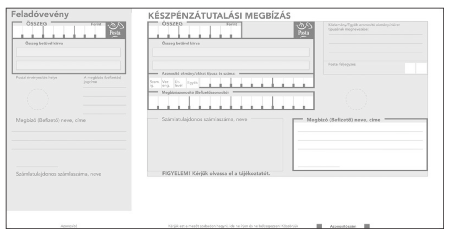Data of the payer to be written on the form (Megbízó (Befizető)'') should have white background, other parts of the cheque should have yellow background. The receipt (Feladóvevény'' on the left) is separated from the rest by a white column. Auxiliary information and the frames are in red. The payer should be able to write in black.

If one writes data in the white region under the label Megbízó (Befizető) neve, címe (Name and address of the payer'') on the right, these data should automatically be copied into the corresponding yellow cells of the receipt on the left.

The actual sum to be transferred is entered using digits in the cell Összeg of the receipt. This number should automatically appear under the other label Összeg on the right.

Upon entering a valid number in the left cell Összeg, your sheet should automatically fill in the fields Összeg betűvel kiírva, that is, the sum should be written out in words as well, according to the grammatical spelling. To achieve this, you may use macros and auxiliary cells, but these should be clearly visible outside the cheque's region.

You can obtain at most 5 points for writing out the numbers in words. If this feature is working, another 5 points can be awarded for accurate graphics.

The spreadsheet (i260.xls, i260.ods, ...) together with a short documentation (i260.txt, i260.pdf, ...) also describing the name and version number of the spreadsheet application should be submitted.

(10 pont)

solution (in Hungarian), statistics

In this exercise you are going to create some figures and 4 web pages illustrating various matchstick puzzles. For creating the web pages, you should use the files forras.txt, 1.jpg, 2.jpg, 3.jpg, 4.jpg and the given example. The compressed file forras.zip containing all these files can be downloaded from our web page.

1. To make the webpage more attractive, use a photo editor and prepare a file csik.jpg by merging the files 1.jpg, 2.jpg, 3.jpg and 4.jpg according to the example.

2. By using a graphics editor, prepare a file abra1.png according to the example and to the matchstick figure on page rejt1.html.

3. Create 4 web pages with names rejtbev.html, rejt1.html, rejt2.html and rejt3.html, and with the following properties:

$\displaystyle a$. The browser's title bar should be Matchstick Puzzles''.

$\displaystyle b$. The background color of the page should be turquoise (code #53868B), while the text color and links should be black all the time.

$\displaystyle c$. Create a $\displaystyle 2\times 2$ table: centered, 800 points wide, and with white background color. Set a 2-point wide frame and a 10-point wide cell margin.

$\displaystyle d$. Cells in the first line of the table should be merged and file csik.jpg should be inserted there.

$\displaystyle e$. The width of the first cell in the second line should be set to 150 points. The cell should contain the following texts: Introduction'', 1st puzzle'', 2nd puzzle'' and 3rd puzzle''. These texts should be converted into links. Your web pages should always contain 3 links. (The text referring back to the page itself should not be a link.) Links should be aligned according to the example.

4. On page rejtbev.html, the text from file forras.txt should appear in the second cell of the second line of the table. Paragraphs should be formatted according to the example.

5. The text Matchstick Puzzles'' should be formatted to Title1'' style and should be centered.

6. The paragraphs describing the rules of the puzzle (Néhány fontos szabály...'') should be listed according to the example and their title should be in bold style.

7. On page rejt1.html, the image from file abra1.png should appear in the second cell of the second line of the table together with the corresponding text from file forras.txt.

8. The remaining two puzzles are not specified here: you should pick those and create the corresponding figures abra2.png and abra3.png for them.

9. The pages rejt2.html and rejt3.html should contain your puzzles and they should be formatted similarly to page rejt1.html.

In the example rejtbev.html, Gyufarejtvény'' is Matchstick Puzzles'', Bevezető'' is Introduction'', while rejtvény'' is puzzle''.

Example: rejtbev.html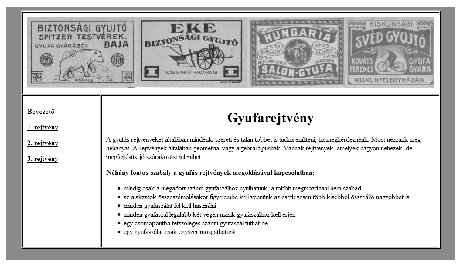rejt1.html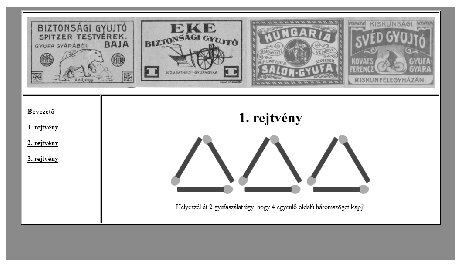All the necessary files and a short documentation should be submitted in a compressed file (i261.zip, i261.rar, ...) also containing the name of the photo editor, graphics editor and web page editor you have used during the solution.

(10 pont)

solution (in Hungarian), statistics## Problems with sign 'S'

Deadline expired on March 10, 2011.

S. 60. On a given square-shaped field we would like to fence off a smaller square with given area so that the little square contains as many trees as possible. The fences should be parallel to the sides of the original square, moreover, if a tree is situated just on the fence, it is considered to be inside''.

Your program should read the area of the original field and that of the little square, further, the position of the trees from the standard input, then give an optimal location for the little square on the standard output.

The first line of the input contains three numbers separated by a space: the side length ($\displaystyle 1 \le N \le 4000$) of the outer square-shaped field, the side length ($\displaystyle 1 \le M \le N$) of the little square to be fenced off, and the number of trees ($\displaystyle 1 \le K \le 1\;000\;000$). Then each of the next $\displaystyle K$ lines specifies the two coordinates (vertical, then horizontal) of a tree ($\displaystyle 0 \le I, J \le N$), separated by a space. Each coordinate is an integer, and no two trees have both coordinates in common.

The first line of the standard output should contain the number of captured trees within the small square, while the second line of the output should have the (vertical, then horizontal) coordinates of the upper left corner of the small square, separated by a space. If there are more solutions, any of them can be presented.

In the example, Példa bemenet'' is a sample input, while Példa kimenet'' is a possible output.

The time limit for your program is 10 seconds in each test case.

The source code and project files of your solution (without the .exe file or any other auxiliary files generated by the compiler) should be submitted together with a short documentation in a compressed folder.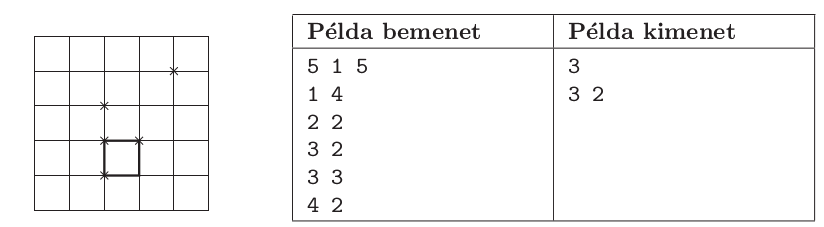(10 pont)

solution (in Hungarian), statistics

\$Var(Body)